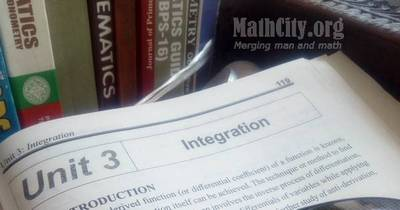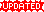# Unit 03: IntegrationNotes (Solutions) of Unit 03: Integration, Calculus and Analytic Geometry, MATHEMATICS 12 (Mathematics FSc Part 2 or HSSC-II), Punjab Text Book Board Lahore. You can view online or download PDF. To view PDF, you must have PDF Reader installed on your system and it can be downloaded from Software section.

• Introduction
• Differentials of Variables
• Distinguishing Between $dy$ and $\delta{y}$
• Simple application of Differentials
• Exercise 3.1
• Integration as anti-derivative ( Inverse of Derivative)
• Some Standrad Formulae for Anti-Derivatives
• Theorems on Anti Derivatives
• Anti-Derivatives of $[f(x)]^n f'(x)$ and $[f(x)]^{-1}f'(x)$
• Exercise 3.2
• Integration by Method of Substitution
• Some Useful Substitutions
• Exercise 3.3
• Integration by parts
• Exercise 3.4
• Integration involving Partial Fraction
• Exercise 3.5
• The Definite Integrals
• The Area Under the Curve
• Theorem of Integrals
• Exercise 3.6
• Application of Definite Integrals
• Exercise 3.7
• Differential Equations
• Solution of a Differential Equation of First Order
• Initial Conditions
• Exercise 3.8
Did you know!
By definition there is no link between derivative and integration of the function.
• Exercise 3.3 | View Online | Download PDF (194KB)• fsc/fsc_part_2_solutions/ch03
• Last modified: 6 months ago
• by Administrator